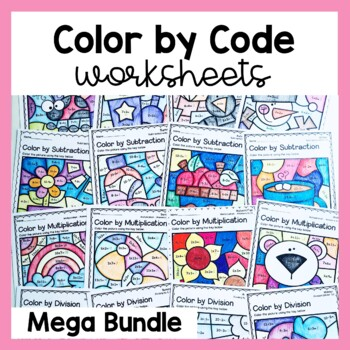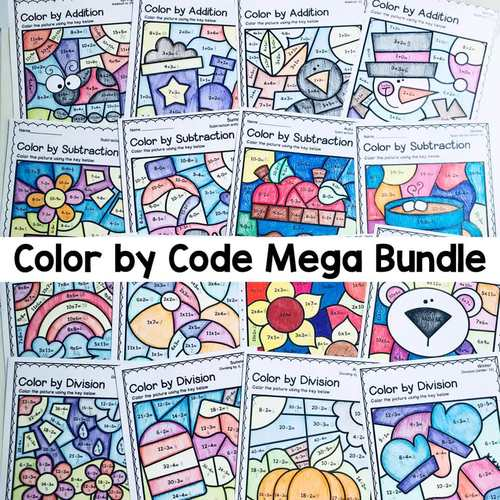# Color by Code Math Worksheets Mega Bundle

Rated 5 out of 5, based on 1 reviews
1 Rating;
2nd - 4th, Homeschool
Subjects
Resource Type
Standards
Formats Included
• Zip
Pages
128 Worksheets + 128 Answer Keys (256 Total)
\$29.99
List Price:
\$48.00
You Save:
\$18.01
Bundle
\$29.99
List Price:
\$48.00
You Save:
\$18.01
Bundle
Report this resource to TPT

#### Products in this Bundle (16)

showing 1-5 of 16 products

### Description

This mega bundle is a selection of color by code / color by number worksheets for addition, subtraction, multiplication, and division. These no prep activities are perfect for your Spring, Summer, Fall, or Winter math lessons and math centers. These activities are perfect for 3rd grade math fact practice.

Students will enjoy solving sums and coloring the mystery pictures in these 16 products!

What is included?

16 products:

• Color by Addition x4 (Spring, Summer, Fall, and Winter)
• Color by Subtraction x4 (Spring, Summer, Fall, and Winter)
• Color by Multiplication x4 (Spring, Summer, Fall, and Winter)
• Color by Division x4 (Spring, Summer, Fall, and Winter)

Each product comes with 8 different mystery pictures and matching answer keys. There are 128 worksheets and 128 answer keys.

Try before you buy! Check out this freebie - Spring Color by Subtraction Worksheet

When/how to use this resource:

• Whole group math lessons
• Small group work (math centers)
• Independent math fact practice
• Early finishers
• Morning work
• Homework
• Easy activity for a sub
• Assessment

Reasons to buy this AMAZING product:

• It’s a time saver – simply print and go!
• It’s a bargain, save 30%!
• It’s versatile – students in many grade levels could try these worksheets, depending on their capabilities
• It’s Common Core aligned
• It’s engaging – student friendly pictures, full of wonderful clip art (which kids will love coloring in)!

Enjoy this resource! Don't forget to follow my store for more awesome products and rate this product for your TPT credits :)

Let's Connect!

Pinterest

Instagram

Looking for more color by codes?

Parts of Speech Color by Code (Grammar Worksheets)

Color by Division Worksheets

Total Pages
128 Worksheets + 128 Answer Keys (256 Total)
Included
Teaching Duration
N/A
Report this resource to TPT
Reported resources will be reviewed by our team. Report this resource to let us know if this resource violates TPT’s content guidelines.

### Standards

to see state-specific standards (only available in the US).
Fluently add and subtract within 1000 using strategies and algorithms based on place value, properties of operations, and/or the relationship between addition and subtraction.
Interpret products of whole numbers, e.g., interpret 5 × 7 as the total number of objects in 5 groups of 7 objects each. For example, describe a context in which a total number of objects can be expressed as 5 × 7.
Interpret whole-number quotients of whole numbers, e.g., interpret 56 ÷ 8 as the number of objects in each share when 56 objects are partitioned equally into 8 shares, or as a number of shares when 56 objects are partitioned into equal shares of 8 objects each. For example, describe a context in which a number of shares or a number of groups can be expressed as 56 ÷ 8.
Determine the unknown whole number in a multiplication or division equation relating three whole numbers. For example, determine the unknown number that makes the equation true in each of the equations 8 × ? = 48, 5 = __ ÷ 3, 6 × 6 = ?.
Apply properties of operations as strategies to multiply and divide. Examples: If 6 × 4 = 24 is known, then 4 × 6 = 24 is also known. (Commutative property of multiplication.) 3 × 5 × 2 can be found by 3 × 5 = 15, then 15 × 2 = 30, or by 5 × 2 = 10, then 3 × 10 = 30. (Associative property of multiplication.) Knowing that 8 × 5 = 40 and 8 × 2 = 16, one can find 8 × 7 as 8 × (5 + 2) = (8 × 5) + (8 × 2) = 40 + 16 = 56. (Distributive property.)